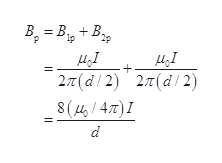Question
22 views

Two very long wires are parallel to each other separated by a distance d.  The same current flows through each wire but in opposite directions.  See the arrows in the diagram.  Let I = 1 Amp and d = 0.1 meter.

a)  What is the direction of the net magnetic field due to the two, long wires in the space between the wires? Explain your answer.

b)  Calculate the magnitude of the net field at point P, which is half-way between the wires.

c)  Calculate the magnitude of the magnetic force per unit length due to wire #1 on #2.

d)  Determine the direction of the force calculated in part (c) and explain your answer.

check_circle

Step 1

(a) The direction of magnetic field due to a current carrying wire is given by Maxwell’s right hand thumb rule.

It states that, if the thumb points in the direction of the current, the curl of the fingers represent the direction of magnetic field.

In the given problem, current flows towards right in the first wire and hence magnetic field between the wires is out of the plane of the paper. Likewise for the second wire, it is out the plane of the paper.

Step 2

(b) Write the expression for magnetic field due to both wires at the midpointhelp_outlineImage TranscriptioncloseВ. — В, μ41 2π(d/2) 2π (d/ ) 3 (14/ 4π) Ι μ.1 d fullscreen
Step 3

Substitute the...

### Want to see the full answer?

See Solution

#### Want to see this answer and more?

Solutions are written by subject experts who are available 24/7. Questions are typically answered within 1 hour.*

See Solution
*Response times may vary by subject and question.
Tagged in
SciencePhysics

### Electromagnetic Induction